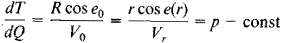Seismic Ray

seismic ray

[′sīz·mik ′rā]
(geology)
The path along which seismic energy travels.

Seismic Ray

a line normal to the front of a seismic wave propagating from the focus of an earthquake. The direction of a ray changes with a change in the velocity of seismic waves along the path of propagation. In a homogeneous, isotropic elastic medium with a constant velocity of wave propagation, a ray is a straight line. In a first approximation for the earth, velocity is taken to be a function of depth; as velocity increases with depth, the paths of the rays become curved, turning convexly downward symmetrically relative to the apexes of the rays. The equation for a ray iswhere T is the ray’s travel time, Q is the epicentral distance in radians, R is the radius of the earth, Vr is the velocity of seismic waves along the ray, e(r) is the angle of inclination of the ray to the horizon at a depth corresponding to the radius r, e0 is the angle of emergence of the seismic ray on the earth’s surface, and V0 is the velocity at the earth’s surface.

Each ray has a deepest point with radius rp. At this point, e = 0, cos e(r) = 1, and p = rp/ Vrp.

I. V. GORBUNOVA

Mentioned in ?
References in periodicals archive ?
Seismic ray tracing using linear traveltime interpolation.
Shortest path calculation of seismic rays. Geophysics, 56(1), 59-67.
Wide-azimuth is an advanced seismic data acquisition technique that gives more refined imaging from the recorded seismic ray paths.
If the measuring base is long, there is indeed much more scope for the shortest "source--sensor" join in a velocity inhomogeneous medium to differ from the seismic ray which propagates along the path of shortest time.
Optimization of a seismic ray tracing with globalization method in anisotropic medium 3D
The azimuth and apparent velocity of P-wave enable us to determine the direction of a seismic ray approaching the array.
Cerveny, V.: 2005, Seismic ray theory, Cambridge University Press.
This method assumes linear propagation of a seismic ray of a direct wave, which is refracted at a distinctive refraction boundary.
The travel times of seismic rays are affected not only by the surface ellipticity bulges at the end of rays but also by the ellipticity of each internal surface of constant seismic velocity encountered by the ray.
Like in optics, seismic energy propagates between two points (the earthquake and the seismograph) along the minimum traveltime path; because of the analogy with optics we often talk about "seismic rays." The travel time anomaly due to the small deviations from the reference-earth model is accumulated along the ray path.
In case of the central seismic source, whose distance from the geophone is 1 to 2 m, one may consider relatively shallow penetration of seismic rays into the rock massif, and the seismic wave then propagates mostly through the disturbed part of the massif.

Site: Follow: Share:
Open / Close